Thursday , August 6 2020
ONLINE BOOK STORE (Shop Now)
Home / Igcse Chemistry Revision Notes / Stoichiometry & The Mole Concept

# Stoichiometry & The Mole Concept

### Writing Ionic Equations:

An ionic equation is a simplified chemical equation that shows the reactions of ionic compounds in water.

Ionic compounds are those compounds, which are soluble in water.

Inorder to write an ionic equation:

• Write the balanced chemical equation of the reaction. Include the state symbols.
• Identify ionic compounds that are soluble in water. These compounds become ions in H20. Rewrite the chemical equation in terms of ions.
• Cancel out the spectator ions (Common).
• Write the ionic equation.

Examples: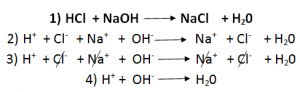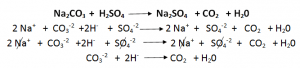### Relative Atomic Mass (Ar):

The mass of an atom compared with the Carbon – 12 atom is called its relative atomic mass.

The mass of one mole of atoms is its “relative  atomic mass” in grams.

### Relative Molecular Mass (Mr):

The mass of a substance made of molecules is known as Relative Molecular Mass.

Hydrogen has (1*2) =   2      and H20 has relative molecular mass of (1*2) + 16 = 18

### Relative Formula Mass (Mr):

The mass of a substance made of ions is known as relative formula mass.  Ammonia (NH3) has Mr of (1*14) + (3*1) =  58.5.

### The Mole:

A mole of a substance is the amount that contains the same number of units as the number of Carbon atoms in 12 grams of carbon-12.

Number of Particles in one mole = 6.02 * 1023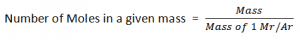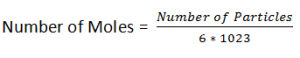### Percentage Compostition of Compounds:

Percentage by mass of an element in a compound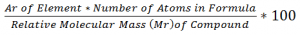Example:

Percentage of Hydrogen in Hydrogen Per Oxide:Water in Coppoer(II) Sulphate:### Emperical Formula:

Emperical Formula of a compound shows:

• Types of elements present in it.
• Simplest Ratio of the different types of atoms in it.

Inorder to Find the Emperical Formula of a compound:

• Write down the each percentage/ mass separately.
• Divide each by their Mr.
• Now divide all of them with one which has the lowest ratio.
• If any one of the answer is in the decimal form, multiply both with any number (lowest) to get make it a whole number

Examples:

A  compound has 40% Carbon 6.6% Hydrogen & 53.3% Oxygen. Calculate the Emperical Formula of the compound.

C                              H                                O

40                            6.6                            53.3

40/12                      6.6/1                          53.3/16

3.33                         6.6                              3.33

3.33/3.33               6.6/3.33                     3.33/3.33

1                              2                                 1

Emperical Formula: CH2O

E.g 2  O.72g of Mg combines with 0.28g of Nitrogen. Find its Emperical Formula.

Mg                               N

0.72                           0.28

0.72/24                      0.28/14

0.03                           0.02

0.03/0.02                  0.02/0.02

1.5                              1

1.5 * 2                        1 * 2

3                                 2

Emperical Formula :Mg3N2

### Molecular Formulae:

The molecular formula shows the actual number of atoms that combine to form a molecule.

To Find the molecular Formula:

E.g. Emperical Formula = HO

Relative Molecular Mass = 34

(H = 1 , O = 16) . Find Molecular Formula?

34/17 =  2

HO * 2  = H202

### Calculating The Volume of  Gas:

1 Mole of every substance occupies 24dm3.

The Concentration of a solution, is the amount of solute in grams or noles, that is dissolved in 1 dm3 of solution.Percentage yield:

Yeild is the amount of product, obtained from a reaction.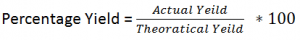Actual Yeild:

It is the amount collected at the end of a chemical reaction. The actual yield is always less than the theoretical yield. It is also known as the Practical Yeild.

Theoratical Yeid:

It is the calculated yield of the amount of prosuct by using stoichiometry. In this Yeild 100% reactants are converted to products, with no losses.

Percentage Purity:

Percentage Purity indicates the amount od pure substance present in a sample of chemical substance.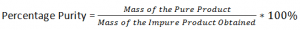Relax your Mind From Studying and WATCH this Beautiful Sunset Painting.

SUBSCRIBE to learn How to Paint## Bases

Bases: Bases are metal oxides and metal Hydroxides. A base is a a substance, that …

1.very usefull

•Very usefull i really hope i will understand more

•Thanks Monica, for appreciating our work.

2.Very easy to understand

•Thanks John,
We hope our Revision Resources have helped you in understanding chemistry.

3.Hello..This is a really nice website. But could you kindly please tell me that from where can I get o’level course Cambridge in Jeddah ,KSA or Karachi,Pakistan. I need it so that I can study online… Please kindly help me….And kindly please add biology notes as well…………. I seriously need your help………….

4.Sarafina Shimuningeni

I struggle alot with chemistry, so I’m really glad I found your page. Thank you so much.

•Thank You Sarafina, We hope that our Revision Resources help you in understanding Chemistry in a much better way.

•Thank you so much. Its really helpful

5.Best notes ever and they are really helpful too

6.Keep publishing more notes.They are really helpful.

7.Erickson ssempebwa

really nice notes
to revise
thank.

•Thank you Erickson, for appreciating our Revision Notes.

8.Thank you admin for your good notes and questions because may help me to answer my chemistry exams. Be blessed

•Thanks Kelvin,

9.Could you add Biology revision notes as well?

•We have noted down your request and will definitely upload biology notes as well.

10.Very very useful!! Excellent!!!

11.I am in love with this website, thanks a lot!